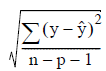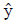# 5.4.5 - Linear Regression Model Evaluation - Teradata Warehouse Miner

## Teradata Warehouse Miner User Guide - Volume 3Analytic Functions

Product
Release Number
5.4.5
Published
February 2018
Language
English (United States)
Last Update
2018-05-04
dita:mapPath
yuy1504291362546.ditamap
dita:ditavalPath
ft:empty
Linear regression model evaluation begins with scoring a table that includes the actual values of the dependent variable. The standard error of estimate for the model is calculated and reported and may be compared to the standard error of estimate reported when the model was built. The standard error of estimate is calculated as the square root of the average squared residual value over all the observations, i.e.whereis the actual value of the dependent variable, is its predicted value, n is the number of observations, and p is the number of independent variables (substituting n-p in the denominator if there is no constant term).